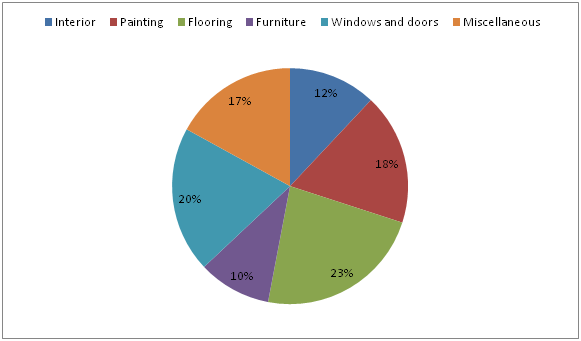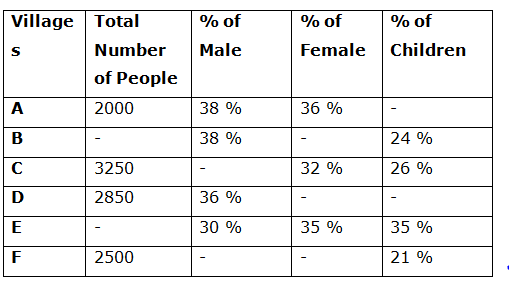# Quantitative Aptitude Questions (Data Interpretation) for NIACL Assistant Mains 2018 Day-180

Dear Readers, IBPS NIACL is conducting Online Examination for the recruitment of Assistant. To enrich your preparation here we have providing new series of Data Interpretation – Quantitative Aptitude Questions. Candidates those who are appearing in NIACL Assistant  Mains Exams can practice these Quantitative Aptitude average questions daily and make your preparation effective.

[WpProQuiz 3429]

Click “Start Quiz” to attend these Questions and view Solutions

Directions (1 – 5): Study the following information carefully and answer the given questions:

The following pie chart shows the percentage distribution of total estimated cost for renovating the house.

Total estimated cost = Rs. 5000001) If the family gets a discount on furniture and pays 15 % less than the estimated cost, then find the amount spent on furniture?

a) Rs. 41000

b) Rs. 43500

c) Rs. 42500

d) Rs. 43000

e) None of these

2) Find the average estimated cost of Interior, Flooring and Furniture together?

a) Rs. 75000

b) Rs. 72000

c) Rs. 78000

d) Rs. 74000

e) None of these

3) Find the ratio of the estimated amount of Interior and painting together to that of the estimated amount of Windows and doors and miscellaneous together?

a) 14 : 19

b) 5 : 7

c) 23 : 29

d) 30 : 37

e) None of these

4) The estimated amount of painting is what percentage more/less than the estimated amount of windows and doors?

a) 10 % more

b) 10 % less

c) 15 % less

d) 15 % more

e) None of these

5) Out of the total estimated cost of furniture, 54 % of the amount is used for showcase and remaining was used for Cupboards and Shelves in the room. Find the difference between the estimated cost for showcase to that of the estimated cost for cupboards and shelves in a room?

a) Rs. 4000

b) Rs. 3500

c) Rs. 4250

d) Rs. 4800

e) None of these

Directions (6 – 10): Study the following information carefully and answer the given questions:

The following table shows the number of people in 6 different villages and the percentage distribution of male, female and children in those villages. Some values are missing.6) Find the ratio between the number of males in village A and C together to that of the number of females together in village B and D together, if the sum of total number of people in village B and C is 5100 and the percentage of female in village D is same as the percentage of female in village B?

a) 1152 : 1113

b) 2178 : 1197

c) 1523 : 4155

d) 2125: 1786

e) None of these

7) Find the difference between the total number of female and children in village D to that of total number of male and female in village F?

a) 178

b) 151

c) 163

d) 139

e) None of these

8) Find the average number of peoples in all the given villages except village F, if the ratio between the total number of people in village A to that of E is 1 : 2 and the total number of female in village B is 703?

a) 2790

b) 3150

c) 2370

d) 2560

e) None of these

9) Total number of children in village C and D together is approximately what percentage of total number of male in village F, if the percentage of female in village D is 38 % and that of village F is 35 %?

a) 130 %

b) 115 %

c) 100 %

d) 144 %

e) 85 %

10) Total number of people in village A and F together is what percentage more/less than the total number of people in village E, if the total number of male in village E is 1200?

a) 15 %

b) 17.5 %

c) 12.5 %

d) 20 %

e) 25 %

Direction (1-5) :

Total estimated cost on furniture = 500000*(10/100) = Rs. 50000

Discount = 15 %

So, the amount spent on furniture = 50000*(85/100) = Rs. 42500

The average estimated cost of Interior, Flooring and Furniture together

= > 500000*[(12 + 23 + 10)/100]*(1/3)

= > Rs. 75000

The estimated amount of Interior and painting together

= > [(12 + 18)/100]*500000

The estimated amount of Windows and doors and miscellaneous together

= > [(20 + 17)/100]*500000

Required ratio = 30 : 37

The estimated amount of painting = 500000*(18/100)

The estimated amount of windows and doors = 500000*(20/100)

Required % = [(20 – 18)/20]*100 = 10 % less

Required difference

= > 500000*(10/100)*(54/100) – 500000*(10/100)*(46/100)

= > 500000*(10/100)*[(54 – 46)/100]

= > 500000*(10/100)*(8/100)

= > Rs. 4000

Direction (6-10) :

The sum of total number of people in village B and C = 5100

The total number of people in village B = 5100 – 3250 = 1850

The percentage of female in village B = 100 – (38 + 24) = 38 %

The percentage of female in village D = 38 %

The number of males in village A and C together

= > 2000*(38/100) + 3250*(42/100)

= > 760 + 1365 = 2125

The number of females together in village B and D together

= > 1850*(38/100) + 2850*(38/100)

= > 703 + 1083 = 1786

Required ratio = 2125: 1786

The total number of female and children in village D

= > 2850*(64/100) = 1824

The total number of male and female in village F

= > 2500*(79/100) = 1975

Required difference = 1975 – 1824 = 151

The ratio between the total number of people in village A to that of E

= 1 : 2

The total number of people in village E = (2000/1)*2 = 4000

The total number of female in village B = 703

= > (38/100)*total number of people in village B = 703

= > Total number of people in village B = 703*(100/38) = 1850

The average number of peoples in all the given villages except village F

= > (2000 + 1850 + 3250 + 2850 + 4000)/5

= > 13950/5 = 2790

The percentage of female in village D = 38 %

The percentage of children in village D = 100 – (36 + 38) = 26 %

The percentage of female in village F = 35 %

The percentage of male in village F = 100 – (35 + 21) = 44 %

Total number of children in village C and D together

= > 3250*(26/100) + 2850*(26/100)

= > 845 + 741 = 1586

The total number of male in village F = 2500*(44/100) = 1100

Required % = (1586/1100)*100 = 144.18 % = 144 %

Total number of people in village A and F together

= > 2000 + 2500 = 4500

Total number of male in village E = 1200

= > (30/100)*total number of people in village E = 1200

= > Total number of people in village E = 1200*(100/30) = 4000

Required % = [(4500 – 4000)/4000]*100 = 12.5 %

Daily Practice Test Schedule | Good Luck

 Topic Daily Publishing Time Daily News Papers & Editorials 8.00 AM Current Affairs Quiz 9.00 AM Quantitative Aptitude “20-20” 11.00 AM Vocabulary (Based on The Hindu) 12.00 PM General Awareness “20-20” 1.00 PM English Language “20-20” 2.00 PM Reasoning Puzzles & Seating 4.00 PM Daily Current Affairs Updates 5.00 PM Data Interpretation / Application Sums (Topic Wise) 6.00 PM Reasoning Ability “20-20” 7.00 PM English Language (New Pattern Questions) 8.00 PM